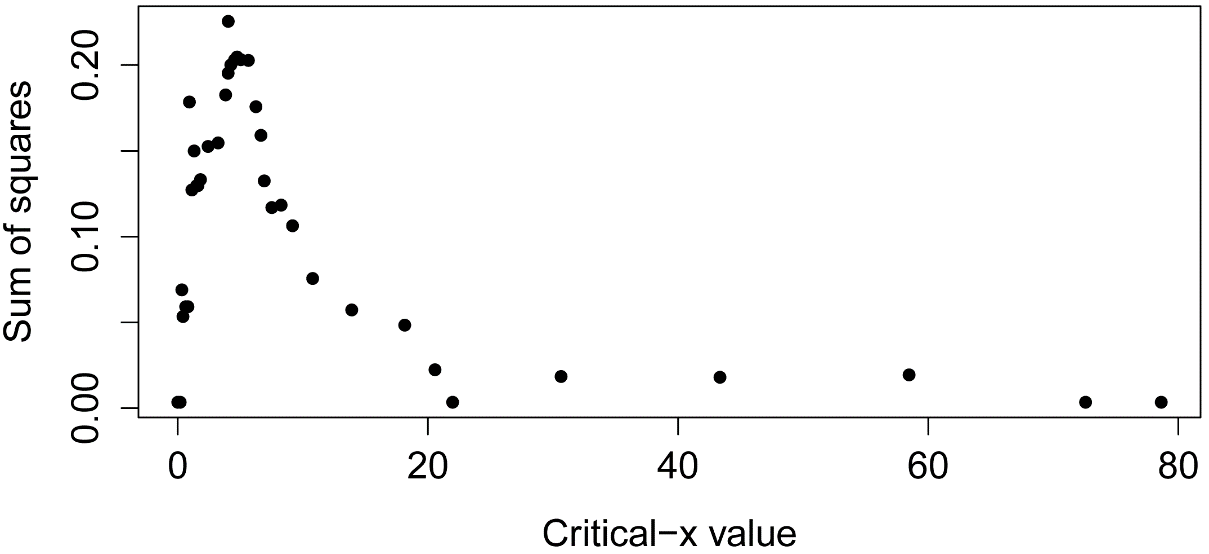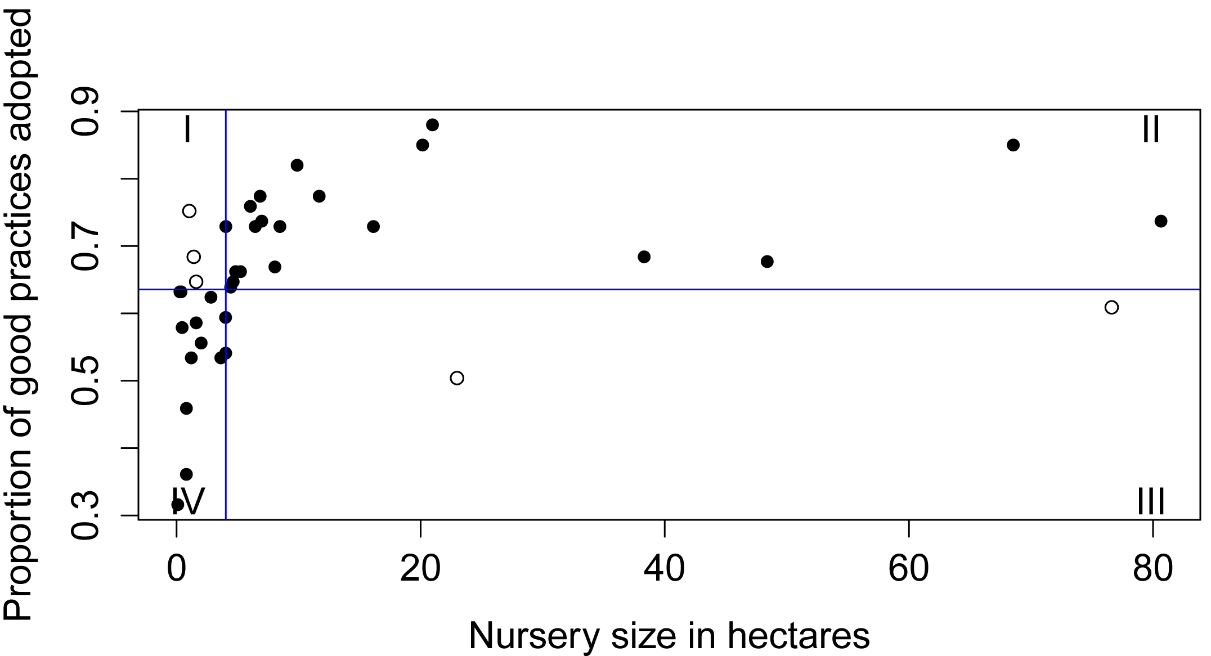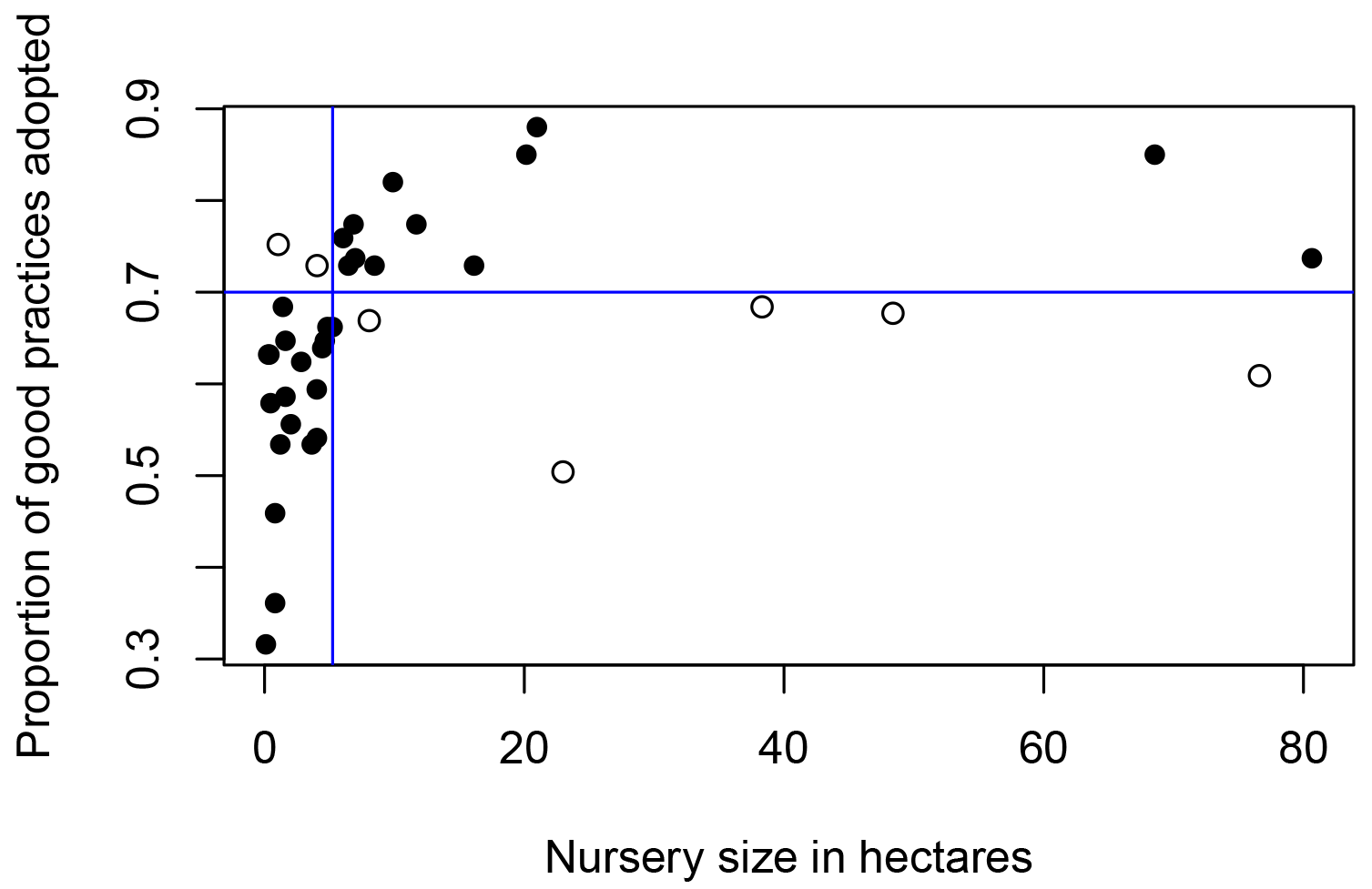## An R Companion for the Handbook of Biological Statistics

Salvatore S. Mangiafico

# Cate–Nelson Analysis

Cate–Nelson analysis is used to divide bivariate data into two groups: one where a change in the x variable is likely to correspond to a change in the y variable, and the other group where a change in x is unlikely to correspond to a change y.  Traditionally this method was used for soil test calibration in agronomy studies.  For example, to determine if a certain level of soil test phosphorus would indicate that adding phosphorus to the soil would likely cause an increase in crop yield or not.

The method can be used for any case in which bivariate data can be separated into two groups, one with a large x variable is associated with a large y, and a small x associated with a small y.  Or vice-versa.

For a fuller description of Cate–Nelson analysis and examples in soil-test and other applications, see Mangiafico (2013) and the references there.

### Custom function to develop Cate–Nelson models

My cateNelson function follows the method of Cate and Nelson (1971).  A critical x value is determined by iteratively breaking the data into two groups and comparing the explained sum of squares of the iterations.  A critical y value is determined by using an iterative process which minimizes the number of data point which fall into Quadrant I and III for data with a positive trend.

Options in the cateNelson function:

·       plotit=TRUE (the default) produces a plot of the data, a plot of the sum of squares of the iterations, a plot of the data points in error quadrants, and a final plot with critical x and critical y drawn as lines on the plot.

·       hollow=TRUE (the default) for the final plot, points in the error quadrants as open circles

·       trend="negative" (not the default) needs to be used if the trend of the data is negative.

·       xthreshold and ythreshold determine how many options the function will return for critical x and critical y.  A value of 1 would return all possibilities.  A value of 0.10 returns values in the top 10% of the range of maximum sum of squares.

·       clx and cly determine which of the listed critical x and critical y the function should use to build the final model.  A value of 1 selects the first displayed value, and a value of 2 selects the second.  This is useful when you have more than one critical x that maximizes or nearly maximizes the sum of squares, or if you want to force the critical y value to be close to some value such as 90% of maximum yield.  Note that changing the clx value will also change the list of critical y values that is displayed.  In the second example I set clx=2 to select a critical x that more evenly divides the errors across the quadrants.

#### Example of Cate–Nelson analysis

##--------------------------------------------------------------------
## Cate-Nelson analysis
## Data from Mangiafico, S.S., Newman, J.P., Mochizuki, M.J.,
##   & Zurawski, D. (2008). Adoption of sustainable practices
##   to protect and conserve water resources in container nurseries
##   with greenhouse facilities. Acta horticulturae 797, 367–372.
##--------------------------------------------------------------------

size =  c(68.55,6.45,6.98,1.05,4.44,0.46,4.02,1.21,4.03,
6.05,48.39,9.88,3.63,38.31,22.98,5.24,2.82,1.61,
76.61,4.64,0.28,0.37,0.81,1.41,0.81,2.02,20.16,
4.04,8.47,8.06,20.97,11.69,16.13,6.85,4.84,80.65,1.61,0.10)

proportion =  c(0.850,0.729,0.737,0.752,0.639,0.579,0.594,0.534,
0.541,0.759,0.677,0.820,0.534,0.684,0.504,0.662,
0.624,0.647,0.609,0.647,0.632,0.632,0.459,0.684,
0.361,0.556,0.850,0.729,0.729,0.669,0.880,0.774,
0.729,0.774,0.662,0.737,0.586,0.316)

library(rcompanion)

cateNelson(x = size,
y = proportion,
plotit=TRUE,
hollow=TRUE,
xlab="Nursery size in hectares",
trend="positive",
clx=1,
cly=1,
xthreshold=0.10,
ythreshold=0.15)

Critical x that maximize sum of squares:

Critical.x.value Sum.of.squares
1            4.035      0.2254775
2            4.740      0.2046979

Critical y that minimize errors:

Critical.y.value Q.i Q.ii Q.iii Q.iv Q.model Q.err Cramer.V
1           0.6355   3   20     2   13      33     5   0.7289
2           0.6430   3   19     3   13      32     6   0.6761
3           0.6470   3   19     3   13      32     6   0.6761
4           0.6545   2   18     4   14      32     6   0.6854
5           0.6620   2   18     4   14      32     6   0.6854
6           0.6015   6   21     1   10      31     7   0.6309
7           0.6280   5   20     2   11      31     7   0.6209
8           0.6320   5   20     2   11      31     7   0.6209

n         = Number of observations
CLx       = Critical value of x
SS        = Sum of squares for that critical value of x
CLy       = Critical value of y
Q         = Number of observations which fall into quadrants I, II, III, IV
Q.Model   = Total observations which fall into the quadrants predicted by the model
p.Model   = Percent observations which fall into the quadrants predicted by the model
Q.Error   = Observations which do not fall into the quadrants predicted by the model
p.Error   = Percent observations which do not fall into the quadrants predicted by the model
Fisher.p  = p-value from Fisher exact test dividing data into these quadrants
Cramer.V  = Cramer's V statistic from dividing data into these quadrants

Final model:

n   CLx        SS    CLy Q.I Q.II Q.III Q.IV Q.Model   p.Model Q.Error
1 38 4.035 0.2254775 0.6355   3   20     2   13      33 0.8684211       5

p.Error Fisher.p.value Cramer.V
0.1315789   8.532968e-06   0.7289Plots showing the results of Cate–Nelson analysis.  In the final plot, the critical x value is indicated with a vertical blue line, and the critical y value is indicated with a horizontal blue line.  Points agreeing with the model are solid, while hollow points indicate data not agreeing with model.  (Data from Mangiafico, S.S., Newman, J.P., Mochizuki, M.J., & Zurawski, D. (2008). Adoption of sustainable practices to protect and conserve water resources in container nurseries with greenhouse facilities. Acta horticulturae 797, 367–372.)

#     #     #

#### Example of Cate–Nelson analysis with negative trend data

##--------------------------------------------------------------------
## Cate-Nelson analysis
## Hypothetical data
##--------------------------------------------------------------------

Input =("
x      y
5     55
7    110
6    120
5    130
7    120
10     55
12     60
11    110
15     50
21     55
22     60
20     70
24     55
")

library(rcompanion)

cateNelson(x = Data\$x,
y = Data\$y,
plotit=TRUE,
hollow=TRUE,
xlab="x",
ylab="y",
trend="negative",
clx=2,      # Normally leave as 1 unless you wish to
cly=1,      # select a specific critical x value
xthreshold=0.10,
ythreshold=0.15)

Critical x that maximize sum of squares:

Critical.x.value Sum.of.squares
1             11.5       5608.974
2              8.5       5590.433
.............

Critical y that minimize errors:

Critical.y.value Q.i Q.ii Q.iii Q.iv Q.model Q.err Cramer.V
1               90   4    1     7    1      11     2   0.6750
2              110   4    1     7    1      11     2   0.6750
3              115   3    0     8    2      11     2   0.6928
4              120   3    0     8    2      11     2   0.6928

n         = Number of observations
CLx       = Critical value of x
SS        = Sum of squares for that critical value of x
CLy       = Critical value of y
Q         = Number of observations which fall into quadrants I, II, III, IV
Q.Model   = Total observations which fall into the quadrants predicted by the model
p.Model   = Percent observations which fall into the quadrants predicted by the model
Q.Error   = Observations which do not fall into the quadrants predicted by the model
p.Error   = Percent observations which do not fall into the quadrants predicted by the model
Fisher.p  = p-value from Fisher exact test dividing data into these quadrants
Cramer.V  = Cramer's V statistic from dividing data into these quadrants

Final model:

n CLx       SS CLy Q.I Q.II Q.III Q.IV Q.Model   p.Model Q.Error
1 13 8.5 5608.974  90   4    1     7    1      11 0.8461538       2

p.Error Fisher.p.value Cramer.V
0.1538462     0.03185703    0.675Plot showing the final result of Cate–Nelson analysis, for data with a negative trend.

#     #     #

### Example of Cate–Nelson analysis with a fixed critical y value

Often when using a Cate–Nelson analysis, we wish to set the critical y at some pre-determined value, and then find the critical x value that best divides the data.  This is common, for example, in agromony studies where we might want to set the critical value at e.g. 90% of maximum potential yield for a crop.

The following example revisits the sustainable nursery practices data above but sets the critical y value at 0.70 (or, 70%).

Here, the first two critical x values in the results (5.24 and 6.05), both result in maximizing the count of observations fitting the model.  It’s possible to sort the resultant data frame by other statistics, like the Pearson chi-square value or the effect size statistics phi.

##--------------------------------------------------------------------
## Data from Mangiafico, S.S., Newman, J.P., Mochizuki, M.J.,
##   & Zurawski, D. (2008). Adoption of sustainable practices
##   to protect and conserve water resources in container nurseries
##   with greenhouse facilities. Acta horticulturae 797, 367–372.
##--------------------------------------------------------------------

size =  c(68.55,6.45,6.98,1.05,4.44,0.46,4.02,1.21,4.03,
6.05,48.39,9.88,3.63,38.31,22.98,5.24,2.82,1.61,
76.61,4.64,0.28,0.37,0.81,1.41,0.81,2.02,20.16,
4.04,8.47,8.06,20.97,11.69,16.13,6.85,4.84,80.65,1.61,0.10)

proportion =  c(0.850,0.729,0.737,0.752,0.639,0.579,0.594,0.534,
0.541,0.759,0.677,0.820,0.534,0.684,0.504,0.662,
0.624,0.647,0.609,0.647,0.632,0.632,0.459,0.684,
0.361,0.556,0.850,0.729,0.729,0.669,0.880,0.774,
0.729,0.774,0.662,0.737,0.586,0.316)

library(rcompanion)

cateNelsonFixedY (x         = size,
y         = proportion,
cly       = 0.70,
plotit    = TRUE,
hollow    = TRUE,
xlab      = "Nursery size in hectares",
ylab      = "Proportion of good practices adopted",
trend     = "positive",
clx       = 1,
outlength = 5,
sortstat  = "error")

Critx Crity Q1 Q2 Q3 Q4 Model Error  N   pQ1   pQ2   pQ3   pQ4  pModel  pError
1   5.24   0.7  2 12  5 19    31     7 38 0.053 0.316 0.132 0.500   0.816   0.184
2   6.05   0.7  2 12  5 19    31     7 38 0.053 0.316 0.132 0.500   0.816   0.184
3   4.84   0.7  2 12  6 18    30     8 38 0.053 0.316 0.158 0.474   0.789   0.211
4   6.45   0.7  3 11  5 19    30     8 38 0.079 0.289 0.132 0.500   0.789   0.211
5   4.64   0.7  2 12  7 17    29     9 38 0.053 0.316 0.184 0.447   0.763   0.237

Fisher.p  Pearson.chisq    Pearson.p      phi
1   0.0001517        12.5500    0.0003972   -0.629

2   0.0001517        12.5500    0.0003972   -0.62
3   0.0005311        10.7500    0.0010420   -0.587
4   0.0007735         9.8400    0.0017080   -0.564
5   0.0018910         9.1610    0.0024730   -0.546Plots showing the final result of Cate–Nelson analysis, for an alysis with a fixed critical y value.

#     #     #

### References

Mangiafico, S.S. 2013. Cate-Nelson Analysis for Bivariate Data Using R-project. Journal of Extension 51:5, 5TOT1. tigerprints.clemson.edu/cgi/viewcontent.cgi?article=2547&context=joe.

Cate, R. B., & Nelson, L.A. (1971). A simple statistical procedure for partitioning soil test correlation data into two classes. Soil Science Society of America Proceedings 35, 658–660.